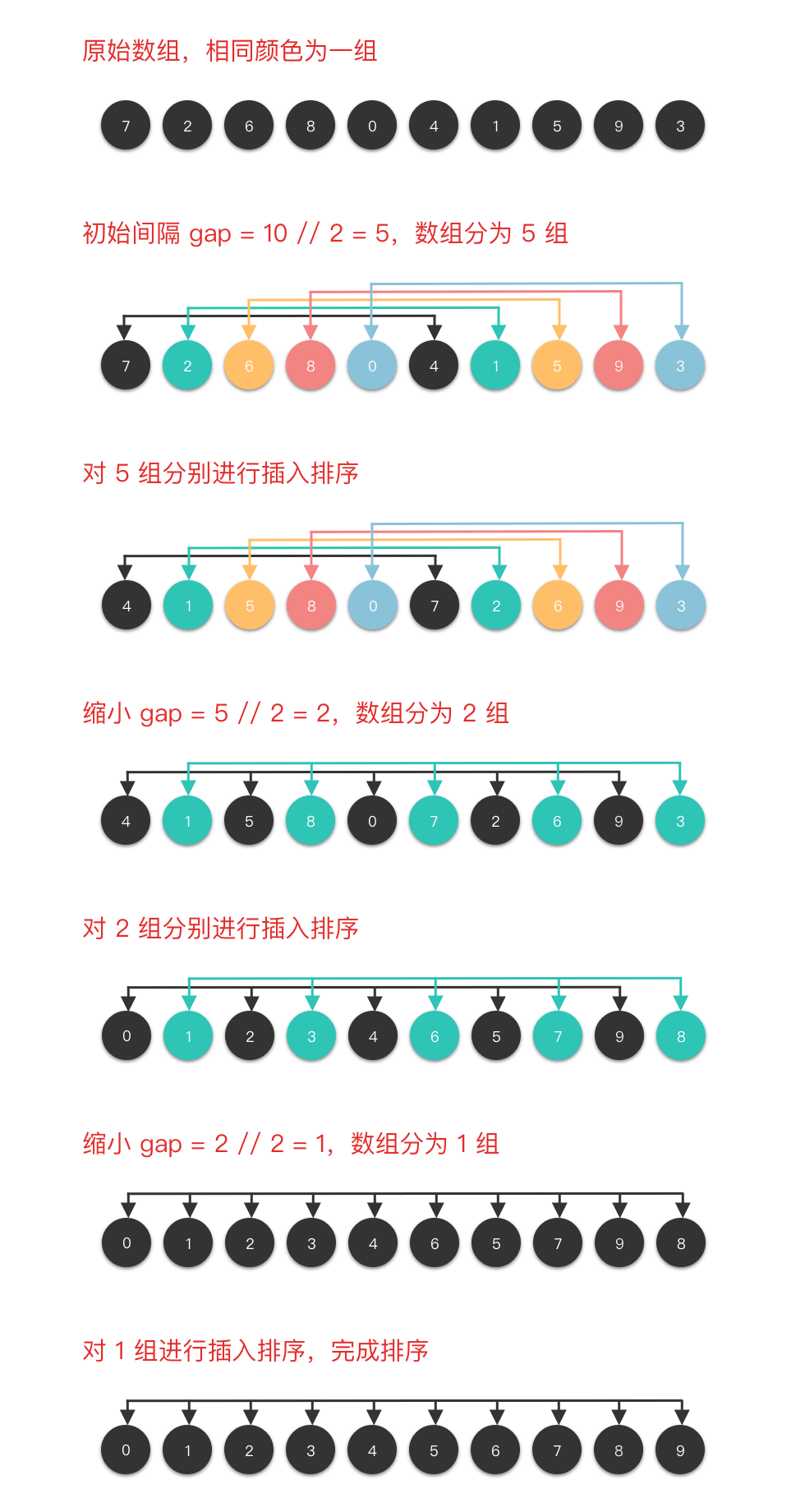04.希尔排序

# 希尔排序 #

## 2. 希尔排序算法步骤 #

1. 确定一个元素间隔数 gap
2. 将参加排序的序列按此间隔数从第 1 个元素开始一次分成若干个子序列，即分别将所有位置相隔为 gap 的元素视为一个子序列。
3. 在各个子序列中采用某种排序算法（例如插入排序算法）进行排序。
4. 减少间隔数，并重新将整个序列按新的间隔数分成若干个子序列，再分别对各个子序列进行排序。依次类推，直到间隔数 gap = 1，排序结束。

## 3. 希尔排序图解演示 ### 4. 希尔排序算法分析 #

• 时间复杂度：介于 $O(n \times \log_2 n)$ 与 $O(n^2)$ 之间。

• 希尔排序方法的速度是一系列间隔数 $gap_i$ 的函数，而比较次数与 $gap_i$ 之间的依赖关系比较复杂，不太容易给出完整的数学分析。
• 由于采用 $gap_i = \lfloor gap_{i-1}/2 \rfloor$ 的方法缩小间隔数，对于具有 $n$ 个元素的序列，若 $gap_1 = \lfloor n/2 \rfloor$，则经过 $p = \lfloor \log_2 n \rfloor$ 趟排序后就有 $gap_p = 1$，因此，希尔排序方法的排序总躺数为 $\lfloor \log_2 n \rfloor$。
• 从算法中也可以看到，最外层的 while 循环为 $\log_2 n$ 数量级，中间层 do-while 循环为 n 数量级。当子序列分得越多时，子序列内的元素就越少，最内层的 for 循环的次数也就越少；反之，当所分的子序列个数减少时，子序列内的元素也随之增多，但整个序列也逐步接近有序，而循环次数却不会随之增加。因此，希尔排序算法的时间复杂度在 $O(n \times \log_2 n)$ 与 $O(n^2)$ 之间。
• 排序稳定性：希尔排序方法是一种 不稳定排序算法

## 5. 希尔排序代码实现 #

  1 2 3 4 5 6 7 8 9 10 11 12 13 14 15 16 17 18 19 20 21 22 23 24  class Solution: def shellSort(self, arr): size = len(arr) gap = size // 2 # 按照 gap 分组 while gap > 0: # 对每组元素进行插入排序 for i in range(gap, size): # temp 为每组中无序序列第 1 个元素 temp = arr[i] j = i # 从右至左遍历每组中的有序序列元素 while j >= gap and arr[j - gap] > temp: # 将每组有序序列中插入位置右侧的元素依次在组中右移一位 arr[j] = arr[j - gap] j -= gap # 将该元素插入到适当位置 arr[j] = temp # 缩小 gap 间隔 gap = gap // 2 return arr def sortArray(self, nums: List[int]) -> List[int]: return self.shellSort(nums)